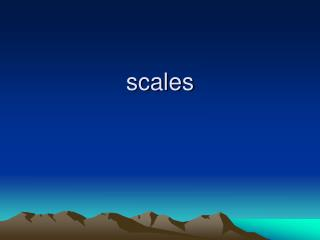DownloadDownload Presentationscales

# scales

Télécharger la présentation## scales

- - - - - - - - - - - - - - - - - - - - - - - - - - - E N D - - - - - - - - - - - - - - - - - - - - - - - - - - -
##### Presentation Transcript

1. scales

2. Which map would you use?

3. Small scale - atlas (large towns in name only) – not detailed • Medium scale - Larger areas (buildings as symbols) • Large scale - city or town maps (defined buildings) – very detailed • The more you use maps the better you will get at recognising the scale and the distance each square represents.

4. Expressing a scale • Centimetres to the kilometre • E.g. 2cm per kilometre. • As a representative fraction • E.g. 1/50000, where you are saying that distances on the map are one fifty thousandth of those on the ground. • As a ratio • E.g. 1:50000 which means for every cm you travel on the map you cover 50000cm on the ground.

5. calculations • A 1:50000 map means: • 1 inch on the map = 50,000 inches on the ground • 1 cm on the map = 50,000 cm on the ground • 1 m on the map = 50,000 m on the ground • Remember that when you are calculating distances each side must be in the same units!

6. Example; if you travelled 5km along a road, how far have you travelled on a map with a scale of 1:50,000? First convert the distance to centimetres 5km =500,000 Next divide the value you get by the ratio 500,000/50,000 =10 On the map you will have travelled 10 cm

7. Example 2 • If you had travelled 2 cm on a 1:50000 map, how far have you travelled on the ground? for every 1 cm you travel on the map you travel 50 000 on the ground so you have travelled 2 x 50000cm 100000cm, which is 1000m or 1km

8. Area

9. Air Photography Photos can be very useful, and in some ways clearer than maps. However they can’t show as large an area.

10. Practice questions • 1. If you measured the distance between two places on a 1:50,000 map and it came to 26 centimetres, what would be the real distance over the ground in Kilometres? • a. 52 • b. 26 • c. 13 • d. 7.5

11. 2. On a 1:50,000 M726 series map, what distance on the ground is represented by 2cm on the map? a. 4Km b. 2Km c. 1Km d. 0.5Km

12. 3. On a 1:50,000 M726 series map, what distance on the ground is represented by one side of a 2cm grid square? • a. 1Km • b. 10Km • c. 0.5Km • d. 5Km

13. 4. For which of the following uses would a map with a scale of 1:1,000,000 be suitable? • a. Showing street and houses in great detail • b. Motoring in the UK • c. Navigating on foot, as in an expedition • d. Long distance aeronautical navigatioin

14. answers • 1. a • 2. c • 3. a • 4. d